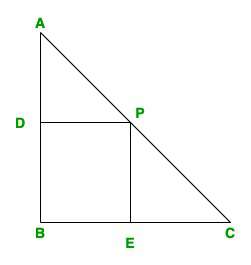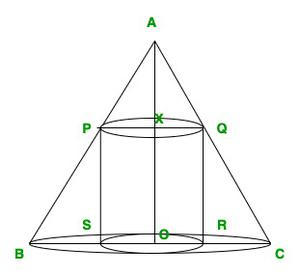# Class 12 NCERT Solutions- Mathematics Part I – Chapter 6 Application of Derivatives – Miscellaneous Exercise on Chapter 6 | Set 2

### Question 12. A point on the hypotenuse of a triangle is at distance a and b from the sides of the triangle. Show that the minimum length of the hypotenuse isSolution:Given, a triangle ABC

Let, PE = a & PD = b

In the △ABC, ∠B = 90

Let ∠C = θ, so, ∠ DPA = θ

DP|| BC.

cosθ = DP/AP = b/AP

AP = b/cosθ

In △EPC,

sinθ = EP/CP = a/CP

CP = a/sin θ

Now AC = h = PA + PC

h =h(θ) = b sec θ + a cosec θ

Put h'(θ) =b sin3θ = a cos 3θ

tan3θ = a/b

tanθ = (a/b)1/3

secθ =cosecθ =hmaxhmax = (b2/3+a2/3)3/2

### (iii) point of inflexion

Solution:

f(x) = (x – 2)4(x + 1)3

On differentiating w.r.t x, we get

f'(x) = 4(x – 2)3(x + 1)3 + 3(x + 1)2(x – 2)4

Put f'(x) = 0

(x – 2)3(x + 1)2 [4(x + 1) + 3(x – 2)] = 0

(x – 2)3(x + 1)2(7x – 2) = 0

Now,

Around x = -1, sign does not change, i.e

x = -1 is a point of inflation

Around x = 2/7, sign changes from +ve to -ve i.e.,

x = 2/7 is a point of local maxima.

Around x = 2, sign changes from -ve to +ve i.e.,

x = 2 is a point of local minima

### Question 14. Find the absolute maximum and minimum values of the function f given by f(x) = cos2 x + sin x, x ∈ [0, π]

Solution:

f(x) = cos2x + sin x; x ϵ [0, π]

On differentiating w.r.t x, we get

f'(x) = 2cos x(-sin x) + cos x = cos x – sin2x

Put f'(x) = 0

cos x(1 – 2sin x) = 0

cos x = 0; sin x = 1/2

In x ϵ[0, π] if cos x = 0, then x = π/2

and if sin x = 1/2, then x = π/6 & 5π/6

Now, f”(x) = -sin x – 2 cos2x

f”(π/2) = -1 + 2 = 1 > 0

x = π/2 is a point of local minima f(π/2) = 1

f”(π/6) =x = π/6 is a point of local maxima f(π/6) = 5/4x = 5π/6​ is a point of local minima f(5π/6) = 5/4

Global/Absolute maxima = ma{f(0), f(π/6), f(π)}

= max{1, 5/4, 1}

= 5/4 = Absolute maxima value

Global/Absolute minima = min{f(0), f(π/2), f(π/6), f(π)}

= min{1, 1, 5/4, 1}

= 1 = Absolute minima value

### Question 15. Show that the altitude of the right circular cone of maximum volume that can be inscribed in a sphere of radius r is 4π/3

Solution:

Let ABC be the cone

and o is the centre of the sphere.

AO = BO = CO = R

AO = h = height of cone

BD = CD = r = radius of cone.

∠DOC = θ                  -(Properties of circle)

In △ DOC,

OD = R cosθ & CD = Rsinθ,

r = R sin θ

AD = AO + OD = R + Rcosθ

h = R(1 + cosθ)

Now, the volume of the cone is

V =v(θ) =Put v(θ) = 0

sinθ[2cosθ + 2cos2θ − sin2θ] = 0

sinθ[2cosθ + 2cos2θ − 1] = 0

sinθ(3cosθ − 1)(1 + cosθ) = 0

sinθ = 0, cos = 1/3​, cosθ = −1

If sinθ = 0, then volume will be 0.

If cosθ = -1, then sinθ = 0 & again volume will be 0.

But if cosθ = 1/3; sinθ = 2√2/3 and

Volume, v = 32/81​πR3, which is maximum.

Height, h = R(1 + cosθ) = R()

h = 4r/3

Hence proved

### Question 16. Let f be a function defined on [a, b] such that f′(x) > 0, for all x ∈ (a, b). Then prove that f is an increasing function on (a, b).

Solution:

Given that on [a, b] f'(x) > 0, for all x in interval I.

So let us considered x1, x2 belongs to I with x1 < x2

To prove: f(x) is increasing in (a, b)

According to the Lagrange’s Mean theorem

f(x2) – f(x1)/ x2 – x1 = f'(c)

f(x2) – f(x1) = f'(c)(x2 – x1)

Where x1 < c < x2

As we know that x1 < x2

so x1 < x2 > 0

It is given that f'(x) > 0

so, f'(c) > 0

Hence, f(x2) – f(x1) > 0

f(x2) < f(x1)

Therefore, for every pair of points x1, x2 belongs to I with x1 < x2

f(x2) < f(x1)

f(x) is strictly increasing in I

### Question 17. Show that the height of the cylinder of maximum volume that can be inscribed in a sphere of radius R is 2R/√3. Also, find the maximum volume.

Solution:

In △ABC,

AC2 = BC2 + AB2

4R2 = 4r2 + h2

r2 = R2……….(1)

Now, volume of cylinder = πr2h

Put the value ov r2 from eq(1), we get

V = π().h

V(h) =On differentiating both side we get

V ‘(h) =Now, put V'(h) = 0

πR2Now the maximum volume of cylinder = π[R2. 2R/√3 – 1/4.4R2/3.2R/√3]

= 4πR3/ 3√3

### Question 18. Show that the height of the cylinder of greatest volume which can be inscribed in a right circular cone of height h and semi-vertical angle α is one-third that of the cone and the greatest volume of the cylinder is 4/27πh3tan2α.

Solution:Let,

XQ = r

XO = h’

AO = h

OC = r’

∠XAQ = α

In triangle AXQ and AOC = XQ/OC = AX/AO

So, r’/r = h-h’/h

hr’ = r(h-h’)

hr’ = rh – rh’

rh’ = rh – hr’

rh’ = h(r – r’)

h’ = h(r – r’)/r

The volume of cylinder = πr’2h’

v = πr’2(h(r – r’)/r)

= π(h(rr’2 – r’3)/r)

On differentiating we get

v’ = πh/r(2rr’ – 3r’2)

Again on differentiating we get

v” = πh/r(2r – 6r’) ………(1)

Now put v’ = 0

πh/r(2rr’ – 3r’2) = 0

(2rr’ – 3r’2) = 0

2r’r = 3r’2

r’ = 2r/3

So, v is maximum at r’ = 2r/3

The maximum volume of cylinder = πh/r[r. 4r2/9 – 8r2/27]

= πhr2[4/27]

= 4/27πh(h tanα)2

= 4/27πh3 tan2α

### Question 19. A cylindrical tank of a radius 10 m is being filled with wheat at the rate of 314 cubic meters per hour. Then the depth of the wheat is increasing at the rate of

(A) 1 m/h      (B) 0.1 m/h      (C) 1.1 m/h      (D) 0.5 m/h

Solution:

Given,

Rate of increase of volume = 314m3/h

ie   dv/dt = 314m3/h

Now, the volume of cylinder = πr2h

v = π.(10)2.h

v = 100πh

On differentiating w.r.t t, we get

dv/dt = 100πSo option A is correct

### Question 20. The slope of the tangent to the curve x = t2 + 3t – 8, y = 2t2 – 2t – 5 at the point (2,– 1) is

(A) 22/7     (B) 6/7     (C) 7/6      (D) -6/7

Solution:

Given that the slope of the tangent to the curve x = t2 + 2t – 8 and y = 2t2 – 2t – 5

On differentiating we getNow, when x = 2,

t2 + 3 – 8 = 2

t2 + 3 – 10 = 0

t2 – 2t + 5t – 10 = 0

(t – 2)(t + 5) = 0

Here, t = 2, t = -5   ……….(1)

When y = -1

2t2 – 2t – 5 = -1

2t2 – 2t – 4 = 0

t2 – t – 2 = 0

(t + 1)(t – 2) = 0

t = -1 or t = 2  ……….(2)

From eq(1) & eq(2) satisfies both,

Now,So, option B is the correct.

### Question 21.The line y = mx + 1 is a tangent to the curve y2 = 4x if the value of m is

(A) 1       (B) 2      (C) 3       (D)1/2

Solution:

The curve if y2 = 4x  …….(1)

On differentiating we getThe slope of the tangent to the given curve at point(x, y)m = 2/y

y = 2/m

The equation of line is y = mx + 1

Now put the value of y, we get the value of x

2/m = mx + 1

x = 2 – m/m

Now put the value of y and x in eq(1), we get

(2/m)2 = 4(2 – m/m)

m = 1

Hence, the option A is correct

### Question 22. The normal at the point (1, 1) on the curve 2y + x2 = 3 is

(A) x + y = 0             (B) x – y = 0

(C) x + y +1 = 0        (D) x – y = 1

Solution:

The equation of curve 2y + x2 = 3

On differentiating w.r.t x, we get

2dy/dx = -x

The slope of the tangent to the given curve at point(1, 1)

dy/dx = -x = -1

m = -1

And slope of normal = 1

Now the equation of normal

(y -1) = 1(x – 1)

x – y = 0

So, B option is correct

### Question 23. The normal to the curve x2 = 4y passing (1, 2) is

(A) x + y = 3            (B) x – y = 3           (C) x + y = 1       (D) x – y = 1

Solution:

The equation of curve is x2 = 4y …….(1)

On differentiating w.r.t x, we get

2x =The slope of normal at (x, y)

-dx/dy = -2/x = m

The slope at given point(1, 2)

m = (y – 2)/(x – 1)

-2/x = (y – 2)/(x – 1)

y = 2/x

Now put the value of y in eq(1)

x2 = 4(2/x)

x = 2

and y = 1

So the point is (2, 1)

Now the slope of normal at point(2, 1) = -2/2 = -1

The equation of the normal is

(y – 1) = -1(x – 2)

x + y = 3

So option A is correct

### (C)(D)Solution:

Given equation 9y2 = x3

On differentiating w.r.t x, we get

18y dy/dx = 3x2

dy/dx = 3x2/18y

dy/dx = x2/6y

Now, the slope of the normal to the given curve at point (x1, y1) isHence, the equation of the normal to the curve at point (x1, y1) isAccording to the question it is given that the normal

make equal intercepts with the axes.

So,…………(1)

The point (x1, y1)lie on the curve,…………(2)

From eq(1) and (2), we getFrom eq(2), we getHence, the required points areSo, option A is correct.

Whether you're preparing for your first job interview or aiming to upskill in this ever-evolving tech landscape, GeeksforGeeks Courses are your key to success. We provide top-quality content at affordable prices, all geared towards accelerating your growth in a time-bound manner. Join the millions we've already empowered, and we're here to do the same for you. Don't miss out - check it out now!